#### Recommend to LibarianSubmit your articleView all articles

Volume 1 Pages 1-71

Volume 2 Pages 1-66

Search this journal

###### How to Cite?
ES Materials & Manufacturing

DOI:10.30919/esmm5f102

ES Materials & Manufacturing, 2018, 1, 27-34

Published online: 05 Sep 2018

Received 17 Jul 2018, Accepted 04 Sep 2018

# Modulated Rate (Time) – Dependent Strain Hardening of Ag/Bi2223 Composite Wire Flattened in a Low – Strength AgMg Alloy Matrix

1 Department of Mechanical Engineering, University of Malaya, 50603 Kuala Lumpur, Malaysia

2SIT Research Laboratories, Shibaura Institute of technology, 3-7-5 Toyosu, Koto-ku, 135-8548 Tokyo, Japan

*E-mail:

## Abstract：

The strain hardening and the pre-compressive forces exerted on the ceramic filaments in Ag/Bi2Sr2Ca2Cu3O10+x(BSCCO or Bi – 2223 in short) composite wire is analysed and discussed in detail.  Tensile stress–strainσε, hardening – relaxation(ε(t)), and the strain – dependent critical current IC(ε)analysis and measurements were carried out on specimen wire at 77 K.  An analysis based on fractal hardening model is presented such that the applied strain starts to exponentially decline at time t=d when normalised σ(t)value equals the value used for the calculation of the composite modulus of elasticity. A biexponential model is further employed over the causally convolved experimental data in order to analyse and compare the relaxation process from both the fractal order and the unnormalised stress range, respectively. Eventually, since the weak metallic part of the composite indicates an abrupt transition from elasticity to direct plasticity, σε data were further analysed by a rate – dependent power – law hardening of the Ramberg – Osgood type. The highest attainable residual strain is therefore used to estimate the intrinsic strain in Bi – 2223 filaments when the pre-compressive stress is completely depleted.

Table of ContentWe use three models to explain the abrupt transition between elastic to plastic behavior in Ag/Bi2223 composite wire and to estimate the intrinsic strain in Bi2223 filaments.

Keywords:Tape; Stress relaxation; Analytical modeling; Mechanical Testing; Sintering

## 1. Introduction

Reliable durability of Ag/Bi2Sr2Ca2Cu3O10+x(BSCCO or Bi – 2223 in short)composite superconductor wires is one of the most challenging design parameters in relation to the environment where these wires are usually employed.  Conventionally, high values of critical transport current Ic, and robust mechanical strain, e capable of weathering some delicate windings and operational routes in solenoid magnet, motor, and generator technologies have to be realised through rigorous laboratory testing prior to actual inclusion of these wires in the manufacturing processes. For instance, Ic is much influenced by densification and how uniform the deformed BSCCO powder is, inside a metal sheath packed with filaments during forming process. In typical powder – in – tube (PIT) process, mechanical deformation step may produce highly densified, homogeneous, textured, and finely oriented BSCCO species. This step is normally followed by thermal treatments for phase transformation and crack healing so that desired grains connectivity is attained. From a well-defined controlled over – pressure sintering technique, (CT – OP), Sato et al1 reported a 30% increase in Ic along the filaments direction in Ag/Bi-2223 wires. The improvement in Ic is ascribed to filament densification, second phase reduction, and voids, pores, and defects elimination from the final product.

On one hand side, intriguing methods have recently been employed to estimate the residual strain in Bi – 2223 filaments of the composite wires.2-5 An in situ synchrotron x – ray technique is used for the estimation of temperature induced residual strain at 77 K.2Elastic constant of the Bi – 2223 phase is measured under tensile load by a defractometer for residual stress analysis, (RESA) and the resulting data is compared with that obtained from a reference sample at RT.3A Bragg peak method is also used to assess the shift in lattice constant of Bi -2223 filaments in a composite wire exposed to bending strain.4 On the other hand, a wide range of values for the modulus of elasticity or the Young’s modulus E, is reported from axial and bending strain experiments in the processed BSCCO rods, filaments, and the overall mechanical response of the composite wire. For example, Passarini et al6 performed a series of single filament measurements on BSCCO wires exhibiting poor and strong transport properties, respectively. They reported a value of E = 121 GPa for the filament extracted from a high Ic wire. Sugano et al7,8 reported the Young’s modulus of about 103 – 106 GPa measured on a Bi2223 composite and, a single filament extracted from VAM BSCCO wires. Oduleye et al9plotted the results for the Young’s modulus of 1 mm length extruded BSCCO rods against their fractional density and found E = 127 GPa when rods were to be densified to zero porosity. In our previous reports,10,11we have shown that E = 129 GPa on a high – strength,  and a high – current wires as a result of densification through consecutive processes of normal sintering, intermediate rolling, and pressure sintering of a Ag/Bi – 2223 composites heat treated at 800 – 835 °C.

It is apparent that the evolution in Ic value through the years has reached more than 300A in Ag/Bi – 2212/2223 wires having tensile stress tolerance in excess of » 500 MPa at 77 and 4.2 K.1,12,13The corresponding improvement in the mechanical strain however is yet to be ascertained in regard to durability in the environment where these wires are intended to be used. Furthermore, relative strain endurance of the metal – sheathed Bi – 2223 filaments to mechanical strain is found to yield scattered results mainly due to the seemingly different processing routes.1-15 Ekin et al15reported the extrinsic effect of irreversible strain limit eirr in the range of 0.20 – 0.35% for Ag/Bi – 2212/2223 at 4.2 K. The report confirmed that the extrinsic nature of eirr can be improved through filaments subdivision and powder diffusion co-processing techniques. Coincidently, from a similar wire such as the one used in this study, Ochiai et al2estimated the thermally induced residual strain in the configuration of Ag/Bi – 2223 composite at 77 K and found an strain tolerance of 0.21% with an intrinsic strain on Bi – 2223 filaments to be » 0.10%.  The strain dependence of bent Bi – 2223 filaments was further considered at room temperature RT by synchrotron X – ray radiation.4It is concluded that the bent filaments suffer asymmetrical effect of both tension and compression which results in less strain endurance on the tensile – strained side of the wire. In contrast, Machiya et al3have found no significant thermal induced residual strain despite the difference in thermal expansion of the composite constituents. The result was attributed to some stress relaxation mechanism associated with the composite processing history. However, in spite of usage of different combinations of advanced experimental and theoretical methods to evaluate the strain tolerance of these batches of Ag/Bi – 2223 wires due to their importance as the germ product of the CT – OP technology, the rate (time) – dependent stress – strain, σ(t)–ε(t) response is not previously considered. In this work, we report the rate (time) – dependent strain hardening in Ag/Bi – 2223 composite wire,10as evaluated from mechanical deformation. Mechanical stress hardening and relaxation were deduced from the monotonsity of σ(t)/ε(t)curves with the help of the strain rate applied during mechanical loading/unloading measurements. We separated the stress – strain,σ(t)/ε(t) responses in order to evaluate the relaxation behaviour of the composite wire at 77 K taking into account the applied mechanical force repercussions on the measured transport current Ic as well as the history of the composite during manufacturing process. We further analyse the experimental findings with a fractal, a biexponential, and a power – low models. Due to low strength metal sheath matrix, the strain hardening property is found to account for the Ramberg – Osgood formalisms.

## 2. Experimental section

Composition of the composite wire under investigation consists of Bi – 2223 oxide filaments surrounded by pure silver whereas the outer sheath material is Ag0.03 wt% Mg alloy. The average volume fraction of the composite constituents, as calculated from Figure 1, are 0.34, 0.33 and 0.33 for Bi – 2223 oxide filaments, pure Ag, and AgMg alloy, respectively. Total number of flattened filaments is 55.  The average width and thickness of the composite wire were 4.2 mm, and 0.22 mm and the average cross – section of this wire was 0.89 mm2.

Tensile stress–strain, σε measurements were carried out using a universal testing machine (Instron – type NMB TCM – 500) at room temperature RT, and at 77 K. Specimen length from Ag/Bi – 2223 composite material was 80 mm with a rubberFigure 1. Cross –sectional geometry for Ag/Bi – 2223 composite wire constituents.

layer of 15 mm attached to each end of the specimen to prevent direct contact with metal grips. The specimen is then inserted between two chuck rods, one of which is motorised. The distance between the chucks was 50 mm. A uniform stroke speed of 0.3 mm min−1 is applied to the wire specimen in the longitudinal direction by the motorised rod. The strain and the strain rate on the specimen were measured by a high resolution couple of Nyilas made extensometers,16 of which the initial gauge length was 25 mm. Monotonic loading/unloading is controlled by an initial strain/stress rate of 1.1 ´ 10– 4 s– 1. Hardening – relaxation is then modeled by treating the stress/strain data by means of Acausal convolution, respectively.17-19Inelastic deformation of the wire is calculated from the load – displacement mode and their time evolution. The critical current measurements and mechanical tests of the specimen were carried out at 77 K with a criterion of 1 μV cm−1 under no external magnetic field.

## 3. Preliminary fractal model

Convolution process is a formal mathematical means to roll – up conditions imposed on a function. When relaxation process of matter contains previous history18,19 present state of relaxation is considered to be normal extension of all previous events that matter has experienced at time τ=0，1,2,...,t.

A general fractional convolution power – law for a function f (t)is thus defined as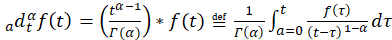(1)

where Г(α)is gamma function. Dynamic time relaxation processes for stress σ(t), and strain ε(t) can generally be convolved using the memory kernel K(t)=ta-1 so that a function Φ(t)will have a convolved derivative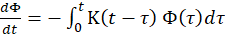.

In a generalised stress – strain relationship,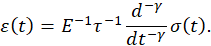Stress relaxation or creep behaviour is given bywith υ=0,a property of elastic solid, and υ=1, a viscous liquid when instantaneous elasticity and long term viscosity were both neglected. κ is a material constant. Henceforth, if a unit step load is uniaxially applied to a specimen from the composite wire for timet=0,and t=b , then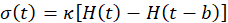(2)

where H is the Heaviside unit step function. Hence, the strain creep response can be written as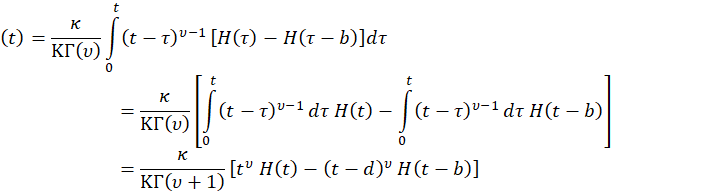(3)

Excluding terms that do not tend to zero as time grows, stress relaxation or creep is defined as the stress required to produce a strain H(t) such that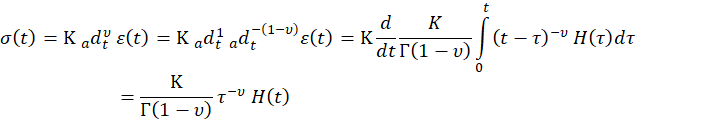(4)

It is worth noting here that when modeling the creep, (retardation), and the relaxation for σ(t) , andε(t)responses, (see Figure 2, red colour curve), normalising conditions for H(t), and δ (t); the Heaviside and the delta functions are acausally convolved based on the  normalised actual data range from experiment.  Note also that exponentυ≈0.2is the value for strain response fractal modeling. Also, the apparent strain relaxation is due mainly to the inability of the soft metal matrix to uptake continuous load after complete fracture of the Bi – 2223 ceramics constituent of the composite wire.Figure 2. Relaxation modulus plotted for the dσ(t)/dε(t)and hardening test data as a function of time. The biexponential model parameters are shown in thelegend. Fractional retardation or creep together with the relaxation curveswere also plotted in this figure using Equations (3) and (4).

## 4. Results and discussion

Figure 2 depicts the relaxation modulus plotted for the σ(t)，andε(t) for hardening modeled data as a function of time. The hardening model is an acausal convolution such  that the relaxation modulus attains a maximum value before exponentially declines. As shown in the figure, for t the composite wire has a retarding creep response. Relaxation period starts and dominate the process when t>b=36s At this particular time, stress is equal to the value used for the calculation of the elastic modulus, E of the composite specimen from the linear elastic portion of the loading/unloading data and the uniaxial σ–ε response. We note from recent literature that the reported E values at RT and 77 K for the likes of this composite wire ranges from 89.2 – 101.0 GPa.2-4  As deduced from the figure, retardation of ceramic filaments damage in Ag/Bi – 2223 composite oppositely encounters the pre-compressive stress applied on the composite during manufacturing stages. This step enhances the overall strength and balances the viscoelastic deformation of the Ag/ Ag – alloy matrix. Application of pre-compressive stress during composite fabrication is intended to result in uniform distribution of residual stress in the filaments, Ag, and Ag – alloy, respectively and, it is these residual stresses that redistribute the total stress exerted on the composite when subjected to incremental tensile load to point of failure. As such, when a uniform tensile stress is applied to a composite specimen mounted on a mechanical actuator, pre-compressive stress release gradually acts to terminate the thermal residual stresses caused by cooling from annealing temperature to RT, and further down to 77 K. In the process of progression of these oppositely competing mechanical and thermal forces, the Ag and Ag – alloy elastically respond todeformation while pre-compressive stress release may act primarily on the Bi – 2223 filaments without much affecting the overall strength of the composite specimen up to its overall elastic limit. Further degradation in the pre-compressive stress with time during hardeningtest is expected to be compensated by strain hardening accompanied by gradual filaments’ fracture accumulation beyond the elastic limit.

Microscopic deformation process in Ag/Bi – 2223 multifilamentary composite may be viewed as follows during gradual imposition and/or continuous tensile stress loading. The initial elastic deformation is reversible since it relates to relative movement of dislocations at atomic scale. The exception here is that microscopic residual stress may be irreversible once occurred in a complex crystalline body such as Bi – 2223 compound because of crystals slip. Cracks in microscopic deformation may grow with the continuation of tensile stress over time and become macroscopic just after the elastic limit is reached, where the first irreversible dislocation in filaments starts to dominate. Conventionally, the stress value at this point is usually taken to be the one that causes a 0.20% permanent strain. It’s reported that the stress hence, the 95% Ic(e) retention in Ag/Bi – 2223 is reversible in the VAMAS tapes up to a strain level of 0.4%.9,17.  Beyond the elastic limit however, microscopic examination of the Ag/Bi – 2212 interface shows no clear shearing or delamination over a permanent strain of 1.0% at 4.2 K.15 In Ag/Bi – 2223 however, the internal strain state of filaments may show partial recovery upon release from a small stress value hence, the resulting Ic(e) partial recovery may generally be due to the presence of reversible factors such as mechanically non-degraded filaments coexisting with microscopically degraded ones.20

In figure 2 we also fit the acuasally convolved experimental σ(t) data using a biexponential least squares model.  This model is used based on the assumption that the relaxation behaviour shown inthe fractal hardeningmodel for ε(t)and in the normal σ(t) curves under continuously applied stress mode is due mainly to different competing responses of the composite constituents. Here we account for the gradual decline of pre – compressive stress, strain hardening, and the consequential growth of microscopic fracture in the Bi – 2223 filaments.

The five– parameter biexponential model is given by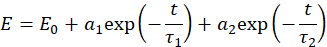(5)

where E0 is offset in the amplitude of the relaxation modulus E at time t, τ1 and τ2 are time decay or relaxation constants, and a1 and a2are amplitude parameters.

To determine the parameters shown in the legend of figure 2, the residuals of the fitting function is taken to be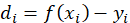(6)

And the weight sum, S of squares of the derivatives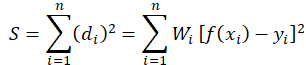(7)

where the weights Wdenote the accuracy of the experimental data.

In order to keep Sat minimum value and, for the determination of amplitude aiparameters, we use S = S  (a1,a2,...,ar)such that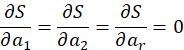Since the amplitude parameters are linear in nature; namely  a1 and a2, Equation (6) may be rewritten as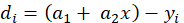(8)

So that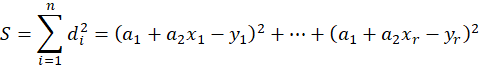when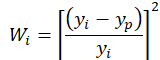.  With yi=Ei, and ypbeing the actual data from experiment, and the predicted data, respectively. n is the number of data points. This gives the percentage difference between the measured and model predicted data points across the whole range of data in equal manner. It follows that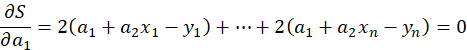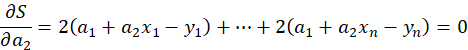,       (9)

Therefore,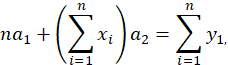,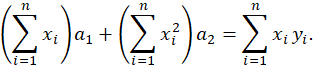(10)

Thus, parameter a1 and a2 are determined and listed together with other model parameters in the legend of figure 2. We note here that there are two distinct time – decaying rates associated with the experimental data.  The τ1and τ2indicate that the relaxation process, whether it was from the hardening – relaxationfractaltest for ε(t) or the acuasally convolved σ(t) data, has separated the decaying effect into two components. This separation may relate to the fact that strain relaxation process in the metallic part of the composite, (Ag and, Ag alloy) is initially delayed by the remainders of a complex mixture of retarding processes provided by the still retarding but mostly broken ceramics filaments.

It is expected that the elastoplastic state of deformation to contain some randomly distributed microcracks along the ceramic core.  When the stress progresses further with time and the corresponding strain, further deformation is naturally stiffened due to mismatch in strain microhardening amongst the grains of composite constituents of ductile Ag, Ag alloy, and the brittle Bi – 2223 ceramic filaments. Therefore, brittle fracture may occur in the filaments when the induced local strain energy from the tensile load equals the energy required to offset the pre-compressive forces. Weak links at grain boundary, lattice defects, and imperfections sites certainly amplify the stress concentration to point of fracture. As indicated by the arrow in figure 3, the first macroscopic crack occurs just after the σ(t) curve changes course towards aFigure 3. σ(t)curve in large strains where two fracture positions were indicated. TheBi – 2223 filaments’ fracture distribution is assumed to correlate withσ(t) = β sin ( πt/Tmax).

horizontal direction. The crack position is identified as the first clear drop in stress magnitude along the direction of strain progression when the stress is fully transmitted to Bi – 2223 filaments.  As shown in figure 3, we assumed that Bi – 2223 filaments’ fracture distribution correlates with σ(t) = β sin ( πt/Tmax), with the constant β≈140, Tmax is the time period used in the experiment, andtis time increment under the condition that 0 ≤ t ≤ Tmax.21 As indicated in the figure, the major crackFigure 4. Stress hardening curve σ(t) for continuous loading. TheIC/(ε) curve is superimposed to show the effect of deformation on transport current in Ag/Bi – 2223 composite wires.

position corresponds to the point where all Bi – 2223 filaments macroscopically break.

This observation, if inspected from figure 4, corresponds to ε=0.30%, a value that falls well beyond the reach of any measurable IC/(ε).  Catastrophic fracture strain,  εf is previously reported2-4 at 0.22% for similar wire. In this work however, at εf =0.20% the IC/(ε) is only 8% remaining from its mechanical strain – free value at 77K.

In figure 4 we show the stress hardening curve as a function of time, σ(t) from continuous loading. We also plot the step-wise σ(ε) curve usually used in the measurements of transport current as a function of strain, IC/(ε)in Ag/Bi – 2223 composite wires.  Both curves show an exact feature at the point of deformation where the stress value becomes nearly constant at σ≈138.5MPa.  The corresponding strain is ε = 0.20%.  Some of the measured  IC/(ε) values are also shown in the figure in order to assess the progress of filaments fracture along the direction of progressive strain.  As indicated by arrowheads, Ic decreases at much slower pace up to σ= 100 MPa which corresponds to ε= 0.15 where the IC/(ε) = 123.54 A.  Change in Ic value up to this point on  σ(ε(t))curves is only less by about 1.46 A from the as – supplied wire which has an external strain – free Ic» 125 A.  This tendency reinforces the assertion that there are no significant fracture in the Bi – 2223 filaments and the very small decrease in IC/(ε) is only caused by few irreversible microscopic residual stresses as a consequence of hardening deformation action against the pre-compressive stress when a continuous or step –wise load is applied.

After heat treatment at high temperatures, the metallic constituents of Ag/Bi – 2223 wire were recrystallized and as a result, pure Ag can be discriminated from Ag alloy by etching away at their boundary. However, the Mg concentration in the AgMg alloy of this wire is only 0.03 wt%, which makes it difficult to draw a clear boundary between Ag and AgMg alloy when etching with NH4OH:H2O2 solution, (see Fig. 1). Therefore, we infer that the added mechanical strength from the alloy part is inferior and as such, two additional observations can be drawn from figures 1, 3, 4, and 5.Figure 5. Normalised stress – strain curves for a matrix with the Ramberg – Osgood hardening.  (a) Solid symbols (¨) indicate an elastic – perfectly plastic flow in the matrix estimated by equation (11). Open symbols (Å) are the strain hardening feature in the composite. Solid line is the Ramberg – Osgood estimation of the hysteretic hardening feature in the composite. (b) Shows the details of the data from the section highlighted by the angled arrows in (a).

First, since the metallic part of the wire consists of low strength AgMg alloy, the σ - ε, and the σ(ε(t))curves follow the Hooke’s law E = σ/ εup to the yield point at about σ≈138.5MPa, where the Bi – 2223 rupture stress occurs at 77 K. The room temperature RT rupture stress in Bi – 2223 filaments of a similar wire is 106 MPa, while that at 77 K is reported to be 135 MPa.13. This persistent rupture stress is immediately followed by strain hardening propagation spanning from ε =0.18%to nearly ε =0.36% after which a relaxation process due mainly to the metallic part responsej to applied load takes place. The strain hardening feature seems to be caused by increase in dislocation density as well as successive breakage of ceramic filaments whereat the metallic part of the composite wire is in plastic deformation state. Beyond  ε =0.36% however, load concentration seems to act mainly on the metallic part due to flow stress hence; the relaxation drop depends on prior history of the Ag/AgMg alloy. As shown in the figures and at a given time, relaxation drop corresponds to the local strain rate sensed by the material and the stress will eventually drop further.

Second, in the original σ - ε, and σ(ε(t))curves of this wire, it’s noted that there is no clear gradual transition from the quasi – elastic region of deformation to the mixed elastoplastic region after which the curve turns horizontal due to plastic deformation. A knee – type transition such as the one observed in this work is previously reported for a low strength Mg and other metallic alloys where the σ - ε curve is shown to follow the Hooke’s law.22. This means that the continuous uptake in  σ - ε curve provides no clear yield point along its path from the quasi – elastic state to the mixed state of elastoplasticity. Henceforth, we assume that the σ(ε(t)) behaviour of the AgMg metal matrix in our Ag/Bi – 2223 composite wire follows a rate – dependent power – law hardening of the Ramberg – Osgood type.  Therefore, σ/σ0 is considered as normalised stress along the linear part of σ - ε curve where the normalising stress, σ0=138.5MPa at 77 K.

Analytical expressions for composite flow behaviour at large strains is derived following Bao and Lin  and others23-25from the Ramberg – Osgood empirical equation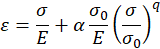(11)

where s is the stress, e0 is the sum of elastic strain,ε0 = σ0/E.  The plastic strain is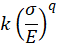with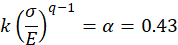. E is the Young’s modulus, qis the strain hardening exponent and,  k is a material constant.

The power – law strain hardening of a unidirectional σ - ε curve is subsequently defined as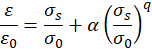(12)

And, the strain rate hardening behaviour of the material is therefore given by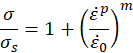(13)

Plastic flow stress occurs atσ=σs=σ0 when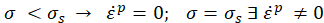.

where εpis the plastic strain rate, ε0is a reference strain rate, and mis the strain rate sensitivity.

In the original work by Ramberg – Osgood22however, combination of Equations (12) and (14) reduces to a dimensionless or normalised  σ - εrelationship according to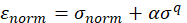(14)

where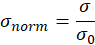, and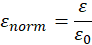. Note that  careful consideration has been made in the selection ofε0 ≈0.15 as a reference strain from the experiment. This is done in order to accommodate the declining effect of pre-compression forces on the filaments due to applied mechanical stress. Indeed, the internal stress state of the filaments comes under direct tensile force when the pre-compressive stress is totally released.26As evidence from figure 4, selection of ε0 ≈0.15 gives a room of about 0.05% strain before the elastic strain limit is exceeded in Bi – 2223 filaments. Degradation of  IC/(ε)  at this strain range further supports this consideration.

In the calculation of the Ramberg – Osgood curves, we use the abovementioned equations together with the volume fraction of Bi – 2223, v=0.34, m=0.35,  initial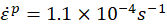, and q=100¥ as parameters. Poisson’s ratio for ceramics is assumed to be ξ=0.30. The results are shown in figures 5 (a), and (b), respectively.  If an elastic to perfect plasticity transition is assumed in the AgMg low – strength alloy matrix of the composite wire then, it’s predictable that the stress – strain behaviour of the matrix will follow the Hooke’s law and then yields indefinitely when the flow stress, σ0 =138.5MPa remains constant.   As represented in figure 5 (a), the behaviour of the metal matrix in this case satisfies equation (11) when the strain hardening exponent q=∞. The composite rate – dependent strain hardening for the flow stress, calculated after normalising the total stress in the composite with σ0=138.5MPa  is plotted against the overall strain normalised by  ε0≈0.15 for q=100as indicated by R – O fit in the figure.  The fitting function produced a hysteretic type strain hardening with a maximum intensity corresponding to 0.36% strain.

In order to show the enormous effect of strain hardening on the composite wire at 77 K, the region highlighted by the angled arrowheads in figure 5(a) is reproduced in detail in figure 5(b).  As shown in the figure, the behaviour of the strain hardening may be due to the increase of dislocation density initiated at the grain boundary before progressing further to form successive rupture in the ceramic filaments. Repetitive increase and decrease of the stress indicates sequential stress transfer between plastically flown matrix and increasingly fracturing filaments, (55 filaments in total). In the ensuing event of continuous hardening, some segmented filaments act as effective strengthening agent to aid the matrix and the stress eventually increases to maximum just before the next filament fractures due which the stress decreases again to minimum. The coercing feature of segmented filaments is seen to have direct effect on the distribution of the strain rate in the matrix as compared with its hardeningless flow curve shown in the figure. This results in a hysteresis form of strain rate distribution in the composite. Exchange in stress carrying capacity between metal matrix and the partially fractured filaments during strain hardening is reported to result from constant stress.27 In this work however, when the stress value responsible for first macroscopic fracture is attained at σ =138.5MPa , the stress immediately decreases ~ 4.0 % from that value before picking up again. It’s apparent that the abrupt transition from elastic to plastic state of the low – strength Ag/AgMg – alloy matrix coupled with concurrent sequential macroscopic fracture formation in the filaments are the main cause of the hysteretic fluctuation of the strain hardening.28

To further assess the strain hardening effect on the composite wire due to increasing stress, we show in figure 6 (a), (b), and (c) the multiple hardening – relaxation curves coupled with the loading/unloading test. A specimen from the composite wire is loaded to σ = 30MPa and then unloaded to zero stress. This step resulted inε = 0.025% . In the second loading/unloading step, the stress is raised from zero to σ = 60MPa before it was unloaded down to σ = 25MPa which corresponds toε = 0.06% , and ε = 0.025%, respectively. A third step is made up to σ = 90MPa  and then resealed down to σ = 25MPa  before the specimen is finally loaded to pointof failure where σ=138.5MPa, ε=0.159% are recorded. As can be seen from figure 6 (c), the Bi – 2223 filaments may respond elastically to the load/unload process because of pre-compressive stress. The highest attainable residual strain in the composite is thus limited byσ=138.5MPa  at ε=0.159% .

Intrinsic strain εintin Bi – 2223 filaments inside the composite wire may be equated by a simple damage strain – like method27,29  through the following expressions.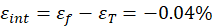(15)

where  εT=0.20% is the aggregate pre-compressive strain estimated from figure 4 according to IC/(ε) profile. εf=0.159%is the fracture strain estimated from the loading/unloading curve shown in figure 6 (c) assuming that  all the 55 Bi – 2223 filaments were mechanically failed  at once at the weakest point in the composite due to severity of the  loading/unloading test, as compared to uniaxial one. Although model and experiment based estimation of the exact value for residual strain may produce satisfactory results, there may still be some uncertainty about it per single filament in a filaments packed long wire. In laboratory samples (typical length≤100mm), if equal cross – section hence, equal pre-compressive stress is assumed then, distribution of stress due to externally applied load must be equal throughout all filaments per specimen effective length regardless of the strength of the alloying material. In this case, all filaments ought to fail at the same point of mechanical failure when the balance of strain hardening against internal pre-compressive stress on them is already zero. As noticed from figure 4, fortunately or unfortunately this is not the case. A subtle residual strain scatter is so results from each experiment.2-14,26–32 Note that the above estimation is a rough one but nonetheless, the achieved σ - ε  values from these tests were useful for describing the strain hardening and the pre-compressive force features of this composite wire.

## 5. Conclusions

Three different model–based methods have been used to assess the strain hardening and the pre-compressive forces exerted on the ceramic filaments in Ag/Bi – 2223 composite wire. Tensile stress–strain,σ - ε, hardening – relaxation σ(ε(t)), and IC/(ε)model and measurements were carried out on specimens from the composite wire. An analysis based on fractal hardening model is controlled such that the applied stress starts to exponentially decline at time t=b when σ(t) value equalled the value used for the calculation of the composite modulus of elasticity from the linear elastic portion of σ - ε curves and the loading/unloading data. A biexponential analysis shows that there are two decaying periods on the relaxation data. In the case that the composite constituents were at elastic state of deformation, these decaying parameters may refer to elastic relaxation in the matrix while the Bi – 2223 filaments relax through length reduction. When the composite experiences plastic deformation however, plastic flow in the low – strength metal matrix accompanied by successive fracture in the ceramic filaments was found.  The strain hardening feature seems to be caused by the increase in dislocation density as well as successive breakage of ceramic filaments whereat the metallic part of the composite wire is in complete plastic flow state.

Since the original σ - ε and σ(ε(t)) curves of the wire indicate no clear gradual transition from the quasi – elastic region of deformation to the mixed elastoplasticity and further to the plastic state, we further treated the experimental data based on the Ramberg – Osgood formalisms. The enormous effect of strain hardening has manifested in a hysteretic form of strain rate hardening distribution over the flow range where the stress on the composite is found to repetitively buckle down to about 4.0%.  The composite rate – dependent strain hardening for flow stress is found to satisfy a strain hardening exponent q=100. The highest attainable residual strain in the composite is thus limited by σ=138.5MPa at ε=0.159%. These values were used to estimate the intrinsic strain the Bi – 2223 filament could withstand at the immediate depletion of pre-compressive stress in the composite.

## Conflict of interest

There are no conflicts to declare.

## References

1. K. Sato, S. Kobayashi, and T. Nakashima, Jpn. J. Appl. Phys., 2012, 51, 010006.
2. S. Ochiai, H. Rokkaku, K. Morishita, J. K. Shin, S. Iwamoto, H. Okuda, M. Hojo, K. Osamura , M. Sato, A. Otto, E. Harely and A. Malozemoff, Supercond. Sci. Technol., 2007, 20, 202-210.
3. S. Machiya, K. Osamura, H. Suzuki, T. Kato, N. Ayai, K. Hayashi and K. Sato, Supercond. Sci. Technol., 2008, 21, 054007.
4. H. Okuda, J. K. Shin, S. Iwamoto, K. Morishita, Y. Mukai, H. Matsubayashi, S. Ochiai, A. Otto, E. J. Harley, A. P. Malozemoff and M. Sato, Scr. Mater., 2008, 58, 687- 690.
5. Z. Zhang, W. Chen and X. Gou, J. Supercond. Novel Magn., 2014, 27, 1387–1396.
6. R. Passerini, M. Dhalle, B. Seeber and R. Flukiger, Supercond. Sci. Technol., 2002, 15, 1507-1511.
7. M. Sugano, K. Osamura and M. Hojo, Supercond. Sci. Technol., 2003, 16, 571-575.
8. M. Sugano, K. Osamura, Physica C, 2004, 402, 341-346.
9. O. Oduleye, S. Penn and McN. N. Alford, Supercond. Sci. Technol., 1998, 11, 858-865.
10. M. I. Adam and K. Osamura, Supercond. Sci. Technol., 2006, 19, 1018-1022.
11. M. I. Adam and K. Osamura, MRS PROC, 2006, 946, HH04-08.
12. A. Otto, Chapter 2.3 “Lamination of Metals onto Bi–2223/Ag Wires” in “Research, Fabrication and Applications of Bi-2223 HTS Wires” World Scientific, 2016.
13. K. Osamura, S. Machiya, H. Suzuki, S. Ochiai, H. Adachi, N. Ayai, K. Hayashi and K. Sato, Supercond. Sci. Technol., 2008, 21, 054010.
14. N. Cheggour, X. Lu, T. Holesinger, T. Stauffer, J. Jiang and L. Goodrich L, Supercond. Sci. Technol., 2012, 25, 015001.
15. J. Ekin, D. Finnemore, Q. Li and C. W. Tenbrink, Appl. Phys. Lett., 1992, 61, 858-860.
16. A. Nyilas, Supercond. Sci. Technol., 2005, 18, 409-415.
17. S. Das, Functional Fractional Calculus, Springer, 2011.
18. A. Krasnobrizha, P. Rozycki, P. Cosson, L. Cornet, communication at 20 International Conference on Composite Materials - ICCM20, Jul 2015, Copenhagen, Denmark, hal-01240728, 14.
19. M. Ostoja-Starzewski and J. Zhang, Fractal Fract., 2018, 2, 12.
20. K. Osamura, M. Sugano and K. Matsumoto, Supercond. Sci. Technol., 2003, 16, 971-975.
21. B. Haken, A. Beuink and H. Kate, IEEE Trans. Appl. Supercond., 1997, 7, 2034-2037.
22. H. Sun, W. Chen and Y. Chen, Physica A, 2009, 388, 4586-4592.
23. W. Ramberg and W. R. Osgood, NACA Technical Note No. 902, 1943.
24. G. Bao and Z. Lin, Acta Mater., 1996, 44, 1011-1019.
25. N. Mostaghel and R. A. Byrd, Int. J. Non Linear Mech., 2002, 37, 1319-1335.
26. L. H. Dai, Z. Ling and Y. L. Bai, Compos. Sci. Technol., 2001, 61, 1057-1063.
27. H. Eck, D. Laan, M. Dhalle, B. Haken and H. Kate, Supercond. Sci. Technol., 2003, 16, 1026-1030.
28. S. Ochiai, H. Okuda, M. Sugano, M. Hojo and K. Osamura, J. Appl. Phys., 2010, 107, 083904.
29. R. Wesche, A. Fuchs, B. Jakob and G. Pasztor, Cryogenics, 1996, 36, 419-426.
30. M. Hojo, M. Hashimoto, M. Tanaka, T. Adachi, M. Sugano, S. Ochiai and K. Osamura, Physica C, 2007, 463-465, 863-866.
31. S. Ochiai, J. Shin, S. Iwamoto, H. Okuda H, S. Oh, D. Ha and M. Sato, J. Appl. Phys., 2008, 103, 123911.
32. X. Gou and Q. Shen, Physica C, 2012, 475, 5-9.